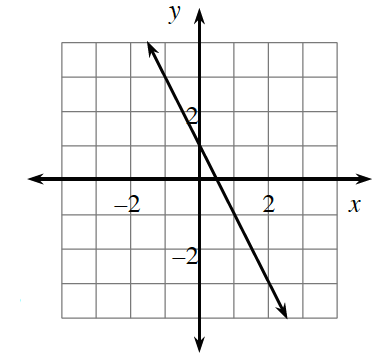### Home > CC3 > Chapter Ch6 > Lesson 6.1.1 > Problem6-3

6-3.

Review what you know about graphs as you complete parts (a) through (d) below.

1. Find the equation of the line graphed at right.

Use the $y$-intercept and the growth rate to write the equation of the line at right.

2. What are its $x$- and $y$-intercepts?

Remember that the $x$-intercept is the point on the graph where $y=0$ and that the $y$-intercept is the point where $x=0$.

3. On your own graph paper, graph the line.

4. On the same set of axes, graph a line that is parallel to the line graphed at right and that goes through the origin $(0,0)$. Find the equation of this new line.

Remember that parallel lines have the exact same growth rate.

Substitute the point given $(0,0)$ into the basic equation
$y=mx+b$ and simplify to find the equation of the line.

$m=$ the growth rate
$b=$ the $y$-interceptExplore the problem using the eTool below.
Click the link at right for the full version of the eTool: 6-3 HW eTool (Desmos)